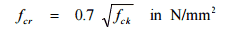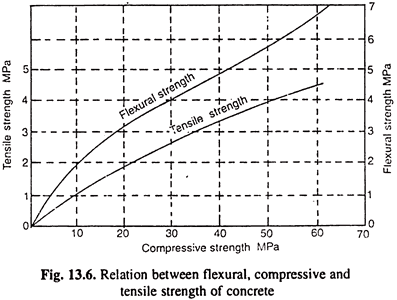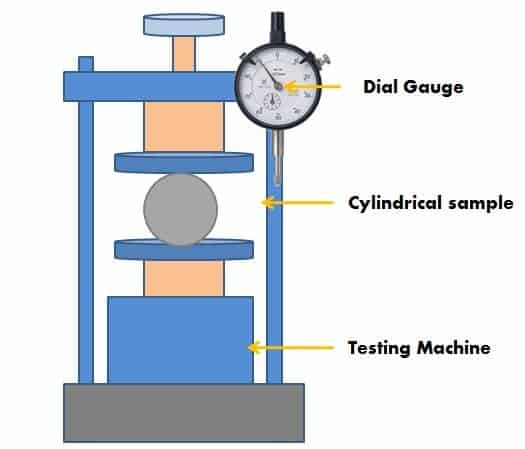# Tensile Strength of Concrete Test: Exploring Split Cylinder Test and Tensile Strength Test Procedure for Concrete Evaluation

Post Contents

## What Is the Tensile Strength of Concrete?

Concrete tensile strength is the ability of concrete to resist tensile force or stress applied to it. The tensile strength of concrete is measured by the split cylinder test of the concrete method. The tensile strength of concrete is measured by the Units of Force per Cross-Sectional area (N/Sq.mm. or Mpa).

As we know that concrete performance in compression is good, but weak in tension force. To counterbalance, this situation reinforcement has been provided in concrete to prevent crack formation.

## Why is Concrete Weak in Tension?

Concrete is not a single solid material like steel which is strong in both tension as well as compression. It is manufactured by mixing cementing materials, water, and aggregate (and sometimes admixtures).Tensile Strength of Concrete Test: Exploring Split Cylinder Test and Tensile Strength Test Procedure for Concrete Evaluation 9

## Interfacial Transition Zone

The “interface transition zone” is the weakest link in the structure. When compressive stress or force is applied to the zone, aggregate transfers the load from one to another. So, concrete in compression does not require much strength to resist compression stresses.

In the case of tensile stresses developed in concrete, the aggregates are trying to pull away from each other so this interfacial transition zone has to bear tensile stresses to hold the whole system together.  Since the strength of this interface zone is weaker than the aggregates, the failure starts at much lower stresses.

As per IS 456:2000, the tensile strength of concrete is given by the equation.Tensile Strength of Concrete Test: Exploring Split Cylinder Test and Tensile Strength Test Procedure for Concrete Evaluation 10Tensile Strength of Concrete Test: Exploring Split Cylinder Test and Tensile Strength Test Procedure for Concrete Evaluation 11

The tensile strength of concrete under direct tension is roughly taken as one-tenth of the strength of concrete under compression.Tensile Strength of Concrete Test: Exploring Split Cylinder Test and Tensile Strength Test Procedure for Concrete Evaluation 12

## Factors Affecting Tensile Strength Concrete

• Quality of Raw Materials – Cement, Sand & Aggregate.
• Water Cement ratio
• Coarse to fine aggregate ratio
• Aggregate cement ratio
• Age of Concrete
• Compaction of concrete
• Temperature
• Relative humidity
• Curing

## Tensile Strength of Concrete Test Methods

For the tensile strength of concrete, we can use the following test methods

1. Uniaxial Tensile Test: This is a direct tension test in which a concrete specimen is held at the ends and pulled apart, inducing uniaxial tensile stress in it. the uniaxial tensile test is one of the most difficult and complicated tests to perfume on concrete but gives the true tensile strength of concrete.
2. Split Cylinder Test: In this test, a concrete cylinder is placed horizontally between loading surfaces and loaded along its diameter. This loading results in producing lateral tensile stress in the cylinder and its splits in tension along its diameter. Refer to ASTM C 496 for the split cylinder test procedure.
3. Flexure Test: In this test, a concrete beam is subjected to four-point loading and loaded to rupture. The specimen cracks due to tensile stresses induced in the bottom fibers under pure bending. This gives the Modulus of Rupture of Concrete. It is performed as per ASTM C78. For the flexure test, another test is available in which load is applied by center point loading as per ASTM C 293. This test also provides flexural strength which will be slightly higher than the 4-point load test.

## Tensile Strength of Concrete Test

The tensile strength of concrete is measured by the split cylinder test of the concrete method and is one of the major properties of concrete, especially in the case of making roads and runways.

The tensile strength of concrete generally varies from 10 % to 12% of its compressive strength.

For determination of the compressive strength of concrete cube, samples are used and for testing tensile strength generally, cylindrical samples are cast.

## Specification of Tensile Strength Test

Specimen

The cylindrical concrete specimen has a diameter of 150 mm and a length of 300mm.

The loading Machine can apply a constant load range of 1.2 MPa/min to 2.4 Mpa/min. (Generally, the test is conducted on the universal testing machine).

Age of Test

The test should be conducted at the age of 7 days and 28 days.

Number of Specimens

For better results comparison at least 3 samples should be tested.

### Apparatus

1. Testing Machine (1.2 Mpa/min to 2.4 Mpa)
2. Tamping Rod (Dia – 16mm, and Length – 600mm)
3. Concrete Mould (Made of Steel)
4. Trowel

### Sample Preparation

• Take a sample from a good-quality mix of concrete.
• Clean the cylindrical mold and ensure the mold is free from dust and rust.
• Fill the mold with concrete in three equal layers with proper compaction with the help of a tamping rod.
• Keep the top surface of the concrete smooth with the trowel.

### Curing

• Mold with concrete samples should be covered with wet gunny bags or kept in undisturbed water for 24 hours at a temperature of 27 ° Celsius ± 2.
• At the end of 24 hours take out the sample and remove the concrete samples from the mold and it should be immersed in the water for 7 or 28 days based on the test.
• Take out a sample from the water after 24 hours prior to the test and it should be in dry condition.

### Tensile Strength Test Procedure

The procedure for split tensile strength of concrete as per is 456 is given below,Tensile Strength of Concrete Test: Exploring Split Cylinder Test and Tensile Strength Test Procedure for Concrete Evaluation 13
1. The bearing surfaces of the machine and the loading strip are free from dust.
2. The specimen should be weighed in Newton prior to the test.
3. Now, place the specimen centrally between the loading strips and ensure the upper platen is parallel to the bottom platen.
4. Then apply the load gradually until the specimen break and note down the Value.
5. The same method has been repeated for other samples

### Tensile Strength of Concrete Formula

The formula for the tensile strength of concrete is given below,Tensile Strength of Concrete Test: Exploring Split Cylinder Test and Tensile Strength Test Procedure for Concrete Evaluation 14

Fct – Tensile Strength of Concrete

P – Maximum load in N/Sqm

L – Length of the Specimen

D – Diameter of the specimen

### Report

The testing report should include the following details,

• Age of the Test
• Date of specimen cast
• The weight of the specimen

## Conclusion

The tensile strength of concrete is a used for assessing its ability to resist tension force and this split cylinder concrete test is mostly used for determining the tensile strength of concrete.

Because concrete exhibits the high compressive strength and relatively tensile strength is low, by determine the strength engineers can ensure that structure such as column, slabs, beams.

The split cylinder test produces and involves careful specimen preparation, proper curing and precise measurement of the splitting load and it allows for accurate calculation of the tensile strength based on the dimension of the specimen

## FAQs

### What is the tensile strength of concrete?

The tensile strength of concrete refers to its ability to resist pulling or stretching forces without breaking. It is an important property to assess the structural integrity and durability of concrete elements.

### What is the split cylinder test of concrete?

The split cylinder test is a common method used to determine the tensile strength of concrete. It involves applying a compressive force to a cylindrical concrete specimen and measuring the resulting tensile splitting force.

### What is the purpose of the split cylinder test?

The split cylinder test is performed to determine the tensile strength of concrete, which is crucial for assessing its structural performance and evaluating its ability to resist cracking and deformation.

### How is the tensile strength of concrete calculated from the split cylinder test?

The tensile strength of concrete is typically calculated using the formula: Tensile Strength = (2 * Maximum Load) / (π * Diameter * Length)

You May Also Like

### Popular Post on Website#### Types of Architects: Exploring the Different Roles and Specializations in Architecture#### Types of Crown Molding: Explore 11 Different Styles and Materials for Elegant Home Décor#### Types of Light Bulbs: A Comprehensive Guide to 50 Different Light Bulb Types, Including Incandescent Bulbs#### 10 Types of Shovels with Pictures for Different Digging Work: A Guide to Choosing the Best Shovels

Scroll to Top